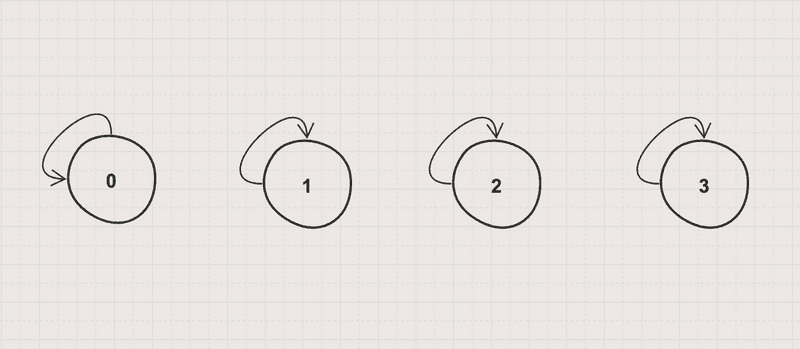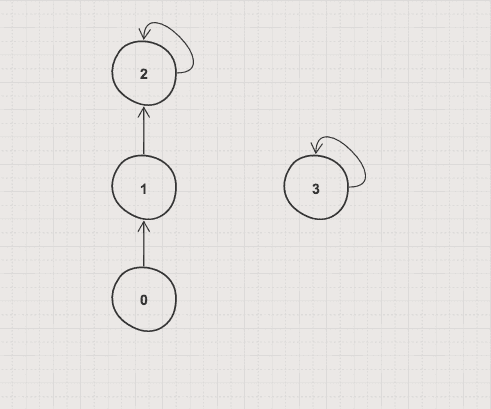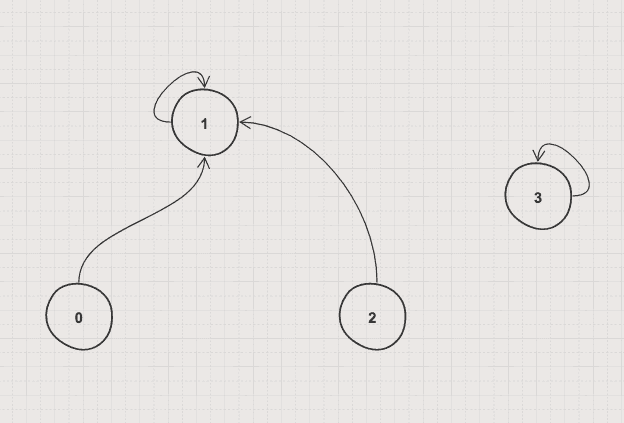# HelloKoding

Practical coding guides

# Disjoint-set Data Structure

Disjoint-sets are sets whose intersection is the empty set. For example, {0, 1, 2} and {3} are disjoint sets, while {0, 1, 2} and {2, 3} are not.

## Problem

• Given some separated elements grouped in disjoint sets
• Find whether 2 elements in the same set or not

## Analysis

• How to determine if 2 elements are in the same set?

Check if they have the same root/representative.

• How to determine the root/representative of a set?

Unionizing 2 elements by a bigger index or by a higher rank

## Approach 1: Union by bigger index

`Find` with compress path and `Union` by bigger index

DisjoinSetUnionByBiggestIndex.java

``````package com.hellokoding.datastructure;

public class DisjoinSetUnionByBiggestIndex {
int[] parents;
int N;

DisjoinSetUnionByBiggestIndex(int N) {
this.N = N;
parents = new int[N];
initDisjoinSet();
}

void initDisjoinSet() {
for (int i = 0; i < N; i++) {
parents[i] = i;
}
}

int findRoot(int x) {
if (parents[x] != x) {
parents[x] = findRoot(parents[x]);
}

return parents[x];
}

void union(int x, int y) {
int rootOfX = findRoot(x);
int rootOfY = findRoot(y);

if (rootOfX == rootOfY) {
return;
}

parents[rootOfX] = rootOfY;
}

public static void main(String[] args) {
int N = 4;
DisjoinSetUnionByBiggestIndex disjoinSet = new DisjoinSetUnionByBiggestIndex(N);
disjoinSet.union(0, 1);
disjoinSet.union(1, 2);

// Check if 1 is in the same set with 2
if (disjoinSet.findRoot(1) == disjoinSet.findRoot(2)) {
System.out.println("1 is in the same set with 2");
} else {
System.out.println("1 is not in the same set with 2");
}

// Check if 1 is in the same set with 3
if (disjoinSet.findRoot(1) == disjoinSet.findRoot(3)) {
System.out.println("1 is in the same set with 3");
} else {
System.out.println("1 is not in the same set with 3");
}
}
}
``````

Initial state of `parents`Final state of `parents` after all unionizing## Approach 2: Union by higher rank

`Find` with compress path and `Union` by higher rank

DisjoinSetUnionByRank.java

``````package com.hellokoding.datastructure;

public class DisjoinSetUnionByRank {
int[] parents, ranks;
int N;

DisjoinSetUnionByRank(int N) {
this.N = N;
parents = new int[N];
ranks = new int[N];
initDisjoinSet();
}

void initDisjoinSet() {
for (int i = 0; i < N; i++) {
parents[i] = i;
}
}

int findRoot(int x) {
if (parents[x] != x) {
parents[x] = findRoot(parents[x]);
}

return parents[x];
}

void union(int x, int y) {
int rootOfX = findRoot(x);
int rootOfY = findRoot(y);

if (rootOfX == rootOfY) {
return;
}

if (ranks[rootOfX] < ranks[rootOfY]) {
parents[rootOfX] = rootOfY;
} else if (ranks[rootOfX] > ranks[rootOfY]) {
parents[rootOfY] = rootOfX;
} else {
parents[rootOfX] = rootOfY;
ranks[rootOfY] = ranks[rootOfY] + 1;
}
}

public static void main(String[] args) {
int N = 4;
DisjoinSetUnionByRank disjoinSet = new DisjoinSetUnionByRank(N);
disjoinSet.union(0, 1);
disjoinSet.union(1, 2);

// Check if 1 is in the same set with 2
if (disjoinSet.findRoot(1) == disjoinSet.findRoot(2)) {
System.out.println("1 is in the same set with 2");
} else {
System.out.println("1 is not in the same set with 2");
}

// Check if 1 is in the same set with 3
if (disjoinSet.findRoot(1) == disjoinSet.findRoot(3)) {
System.out.println("1 is in the same set with 3");
} else {
System.out.println("1 is not in the same set with 3");
}
}
}
``````

Initial state of `parents`Final state of `parents` after all unionizing